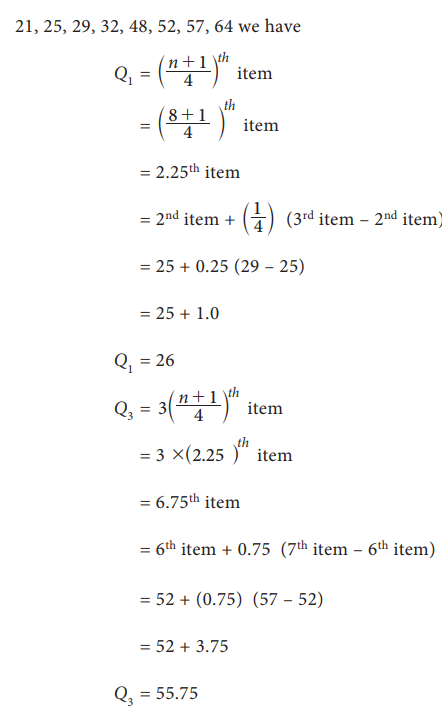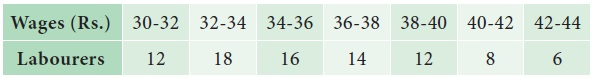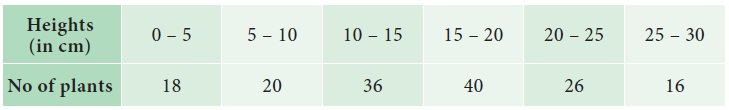Home | | Statistics 11th std | Partition Measures

# Partition Measures

1. Quartiles, 2.Deciles 3. Percentiles

Partition Measures

## Quartiles

There are three quartiles denoted by Q1, Q2 and Q3 divides the frequency distribution in to four equal partsThat is 25 percent of data will lie below Q1, 50 percent of data below Q2 and 75 percent below Q3. Here Q2 is called the Median. Quartiles are obtained in almost the same way as median

### Quartiles for Raw or Ungrouped data:

If the data set consist of n items and arranged in ascending order then### Example 5.30

Compute Q1 and Q3 for the data relating to the marks of 8 students in an examination given below 25, 48, 32, 52, 21, 64, 29, 57

### Solution:

n = 8

Arrange the values in ascending order

21, 25, 29, 32, 48, 52, 57, 64 we haveQuartiles for Discrete Series (grouped data)

Step 1: Find cumulative frequencies

Step 2 : Find ((N+1)/ 4)

Step 3 : See in the cumulative frequencies, the value just greater than ((N+1)/ 4) the corresponding value of x is Q1

Step 4 : Find 3((N+1)/ 4)

Step 5 : See in the cumulative frequencies, the value just greater than 3((N+1)/ 4) then the corresponding value of x is Q3.

### Example 5.31

Compute Q1 and Q3 for the data relating to age in years of 543 members in a village### Solution:## Quartiles for Continuous series (grouped data)

Step 1: Find cumulative frequencies

Step 2 : Find (N/4)

Step 3 : Q1 class is the class interval corresponding to the value of the cumulative frequency just greater than (N/4)

Step 4 : Q3 class is the class interval corresponding to the value of the cumulative frequency just greater than 3 (N/4)### Example 5.32

Calculate the quartiles Q1 and Q3 for wages of the labours given below### Solution:## Deciles

Deciles are similar to quartiles. Quartiles divides ungrouped data into four quarters and Deciles divide data into 10 equal parts .

### Example 5.33

Find the D6 for the following data

11, 25, 20, 15, 24, 28, 19, 21

### Solution:

Arrange in an ascending order

11,15,19,20,21,24,25,28### Example 5.34

Calculate D5 for the frequency distribution of monthly income of workers in a factory### Solution:## Percentiles

The percentile values divide the frequency distribution into 100 parts each containing 1 percent of the cases. It is clear from the definition of quartiles, deciles and percentiles

Relationship

P25 = Q1

P50 = Median = Q2

P75 = 3rd quartile = Q3

### Example 5.35

The following is the monthly income (in 1000) of 8 persons working in a factory. Find P30 income value

10,14, 36, 25, 15, 21, 29, 17

### Solutions:

Arrange the data in an ascending order.

n = 8

10,14,15,17,21,25,29,36### Example 5.36

Calculate P61 for the following data relating to the height of the plants in a garden### Solution:Tags : Formula, Solved Example Problems | Statistics , 11th Statistics : Chapter 5 : Measures of Central Tendency
Study Material, Lecturing Notes, Assignment, Reference, Wiki description explanation, brief detail
11th Statistics : Chapter 5 : Measures of Central Tendency : Partition Measures | Formula, Solved Example Problems | Statistics